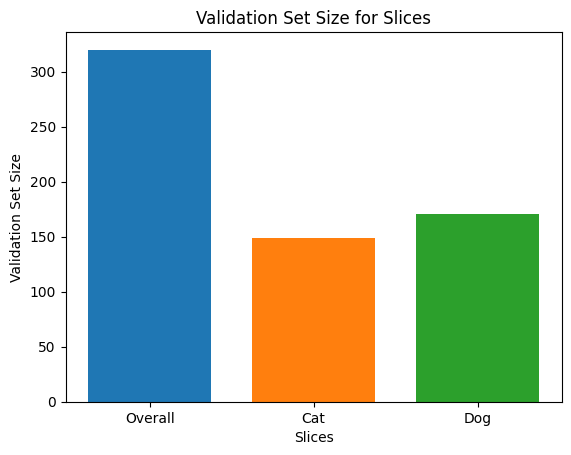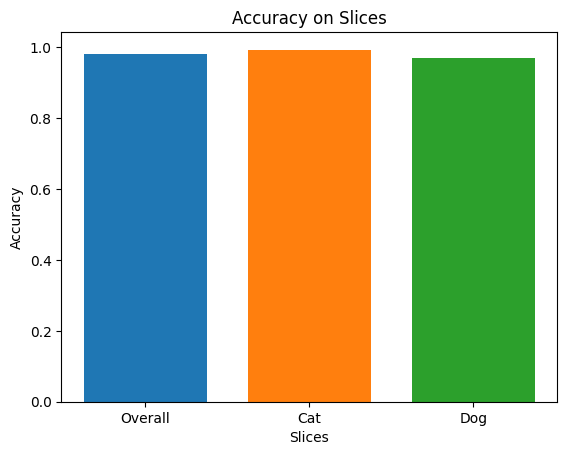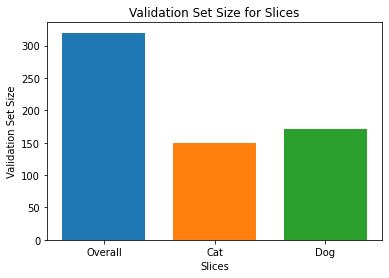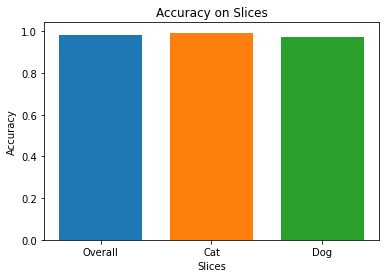Google I/O is a wrap! Catch up on TensorFlow sessions

# Standalone Model Card Toolkit Demo

This "standalone" notebook demonstrates using the Model Card Toolkit without the TFX/MLMD context. To learn how to use Model Card Toolkit with TFX/MLMD, please check MLMD Model Card Toolkit Demo.

## Objective

We are using a Keras model in this demo. But the logic below also applies to other ML frameworks in general.

## Setup

We first need to a) install and import the necessary packages, and b) download the data.

### Upgrade to Pip 20.2 and install the Model Card Toolkit

````pip install --upgrade pip==21.3`
`pip install 'model-card-toolkit>=1.0.0,<1.1'`
`pip install 'tensorflow>=2.3.1'`
```

#### Did you restart the runtime?

If you are using Google Colab, the first time that you run the cell above, you must restart the runtime (Runtime > Restart runtime ...). This is because of the way that Colab loads packages.

### Imports

``````import tensorflow as tf
import numpy as np
import model_card_toolkit as mctlib
from model_card_toolkit.documentation.examples import cats_vs_dogs
from model_card_toolkit.utils.graphics import figure_to_base64str
import tempfile
import matplotlib.pyplot as plt
from IPython import display
import requests
import os
import zipfile
``````
```2022-04-27 09:21:26.163529: W tensorflow/stream_executor/platform/default/dso_loader.cc:59] Could not load dynamic library 'libcudart.so.10.1'; dlerror: libcudart.so.10.1: cannot open shared object file: No such file or directory
```

## Model

We will use a pretrained model with architecture based off MobileNetV2, a popular 16-layer image classification model. Our model has been trained to distinguish between betweens cats and dogs using the Cats vs Dogs dataset. The model training was based on the TensorFlow transfer learning tutorial.

``````URL = 'https://storage.googleapis.com/cats_vs_dogs_model/cats_vs_dogs_model.zip'
BASE_PATH = tempfile.mkdtemp()
ZIP_PATH = os.path.join(BASE_PATH, 'cats_vs_dogs_model.zip')
MODEL_PATH = os.path.join(BASE_PATH,'cats_vs_dogs_model')

r = requests.get(URL, allow_redirects=True)
open(ZIP_PATH, 'wb').write(r.content)

with zipfile.ZipFile(ZIP_PATH, 'r') as zip_ref:
zip_ref.extractall(BASE_PATH)

``````
```2022-04-27 09:21:29.907930: W tensorflow/stream_executor/platform/default/dso_loader.cc:59] Could not load dynamic library 'libcudart.so.10.1'; dlerror: libcudart.so.10.1: cannot open shared object file: No such file or directory
2022-04-27 09:21:29.908050: W tensorflow/stream_executor/platform/default/dso_loader.cc:59] Could not load dynamic library 'libcublas.so.10'; dlerror: libcublas.so.10: cannot open shared object file: No such file or directory
2022-04-27 09:21:29.909596: W tensorflow/stream_executor/platform/default/dso_loader.cc:59] Could not load dynamic library 'libcusolver.so.10'; dlerror: libcusolver.so.10: cannot open shared object file: No such file or directory
2022-04-27 09:21:29.909667: W tensorflow/stream_executor/platform/default/dso_loader.cc:59] Could not load dynamic library 'libcusparse.so.10'; dlerror: libcusparse.so.10: cannot open shared object file: No such file or directory
2022-04-27 09:21:29.909735: W tensorflow/stream_executor/platform/default/dso_loader.cc:59] Could not load dynamic library 'libcudnn.so.7'; dlerror: libcudnn.so.7: cannot open shared object file: No such file or directory
2022-04-27 09:21:29.909743: W tensorflow/core/common_runtime/gpu/gpu_device.cc:1753] Cannot dlopen some GPU libraries. Please make sure the missing libraries mentioned above are installed properly if you would like to use GPU. Follow the guide at https://www.tensorflow.org/install/gpu for how to download and setup the required libraries for your platform.
Skipping registering GPU devices...
```

## Dataset

In the cats-vs-dogs dataset, label=0 corresponds to cats while label=1 corresponds to dogs.

``````def compute_accuracy(data):
x = np.stack(data['examples'])
y = np.asarray(data['labels'])
_, metric = model.evaluate(x, y)
return metric
``````
``````examples = cats_vs_dogs.get_data()
print('num validation examples:', len(examples['combined']['examples']))
print('num cat examples:', len(examples['cat']['examples']))
print('num dog examples:', len(examples['dog']['examples']))
``````
```num validation examples: 320
num cat examples: 149
num dog examples: 171
2022-04-27 09:21:41.176761: W tensorflow/core/kernels/data/cache_dataset_ops.cc:798] The calling iterator did not fully read the dataset being cached. In order to avoid unexpected truncation of the dataset, the partially cached contents of the dataset will be discarded. This can happen if you have an input pipeline similar to `dataset.cache().take(k).repeat()`. You should use `dataset.take(k).cache().repeat()` instead.
```
``````accuracy = compute_accuracy(examples['combined'])
cat_accuracy = compute_accuracy(examples['cat'])
dog_accuracy = compute_accuracy(examples['dog'])
``````
```10/10 [==============================] - 1s 68ms/step - loss: 0.0794 - binary_accuracy: 0.9812
5/5 [==============================] - 0s 55ms/step - loss: 0.0608 - binary_accuracy: 0.9933
6/6 [==============================] - 0s 55ms/step - loss: 0.0956 - binary_accuracy: 0.9708
```

## Use the Model Card Toolkit

### Initialize the Model Card Toolkit

The first step is to initialize a `ModelCardToolkit` object, which maintains assets including a model card JSON file and model card document. Call `ModelCardToolkit.scaffold_assets()` to generate these assets and return a `ModelCard` object.

``````# https://github.com/tensorflow/model-card-toolkit/blob/master/model_card_toolkit/model_card_toolkit.py
model_card_dir = tempfile.mkdtemp()
mct = mctlib.ModelCardToolkit(model_card_dir)

# https://github.com/tensorflow/model-card-toolkit/blob/master/model_card_toolkit/model_card.py
model_card = mct.scaffold_assets()
``````

### Annotate the Model Card

The `ModelCard` object returned by `scaffold_assets()` has many fields that can be directly modified. These fields are rendered in the final generated Model Card document. For a comprehensive list, see model_card.py. See the documentation for more details.

#### Text Fields

##### Model Details

`model_card.model_details` contains many basic metadata fields such as `name`, `owners`, and `version`. You can provide a description for your model in the `overview` field.

``````model_card.model_details.name = 'Fine-tuned MobileNetV2 Model for Cats vs. Dogs'
model_card.model_details.overview = (
'This model distinguishes cat and dog images. It uses the MobileNetV2 '
'architecture (https://arxiv.org/abs/1801.04381) and is trained on the '
'Cats vs Dogs dataset '
'(https://www.tensorflow.org/datasets/catalog/cats_vs_dogs). This model '
'performed with high accuracy on both Cat and Dog images.'
)
model_card.model_details.owners = [
]
model_card.model_details.version = mctlib.Version(name='v1.0', date='08/28/2020')
model_card.model_details.references = [
mctlib.Reference(reference='https://www.tensorflow.org/guide/keras/transfer_learning'),
mctlib.Reference(reference='https://arxiv.org/abs/1801.04381'),
]
model_card.model_details.citations = [mctlib.Citation(citation='https://github.com/tensorflow/model-card-toolkit/blob/master/model_card_toolkit/documentation/examples/Standalone_Model_Card_Toolkit_Demo.ipynb')]
``````
##### Quantitative Analysis

`model_card.quantitative_analysis` contains information about a model's performance metrics.

Below, we create some synthetic performance metric values for a hypothetical model built on our dataset.

``````model_card.quantitative_analysis.performance_metrics = [
mctlib.PerformanceMetric(type='accuracy', value=str(accuracy)),
mctlib.PerformanceMetric(type='accuracy', value=str(cat_accuracy), slice='cat'),
mctlib.PerformanceMetric(type='accuracy', value=str(dog_accuracy), slice='Dog'),
]
``````
##### Considerations

`model_card.considerations` contains qualifying information about your model - what are the appropriate use cases, what are limitations that users should keep in mind, what are the ethical considerations of application, etc.

``````model_card.considerations.use_cases = [
mctlib.UseCase(description='This model classifies images of cats and dogs.')
]
model_card.considerations.limitations = [
mctlib.Limitation(description='This model is not able to classify images of other classes.')
]
model_card.considerations.ethical_considerations = [mctlib.Risk(
name=
'While distinguishing between cats and dogs is generally agreed to be '
'a benign application of machine learning, harmful results can occur '
'when the model attempts to classify images that don’t contain cats or '
'dogs.',
mitigation_strategy=
'Avoid application on non-dog and non-cat images.'
)]
``````

#### Graph Fields

It's often best practice for a report to provide information on a model's training data, and its performance across evaluation data. Model Card Toolkit allows users to encode this information in visualizations, rendered in the Model Card.

`model_card` has three sections for graphs -- `model_card.model_parameters.data.train.graphics` for training dataset statistics, `model_card.model_parameters.data.eval.graphics` for evaluation dataset statistics, and `model_card.quantitative_analysis.graphics` for quantitative analysis of model performance.

Graphs are stored as base64 strings. If you have a matplotlib figure, you can convert it to a base64 string with `model_card_toolkit.utils.graphics.figure_to_base64str()`.

``````# Validation Set Size Bar Chart
fig, ax = plt.subplots()
width = 0.75
rects0 = ax.bar(0, len(examples['combined']['examples']), width, label='Overall')
rects1 = ax.bar(1, len(examples['cat']['examples']), width, label='Cat')
rects2 = ax.bar(2, len(examples['dog']['examples']), width, label='Dog')
ax.set_xticks(np.arange(3))
ax.set_xticklabels(['Overall', 'Cat', 'Dog'])
ax.set_ylabel('Validation Set Size')
ax.set_xlabel('Slices')
ax.set_title('Validation Set Size for Slices')
validation_set_size_barchart = figure_to_base64str(fig)
````````````# Acuracy Bar Chart
fig, ax = plt.subplots()
width = 0.75
rects0 = ax.bar(0, accuracy, width, label='Overall')
rects1 = ax.bar(1, cat_accuracy, width, label='Cat')
rects2 = ax.bar(2, dog_accuracy, width, label='Dog')
ax.set_xticks(np.arange(3))
ax.set_xticklabels(['Overall', 'Cat', 'Dog'])
ax.set_ylabel('Accuracy')
ax.set_xlabel('Slices')
ax.set_title('Accuracy on Slices')
accuracy_barchart = figure_to_base64str(fig)
``````Now we can add them to our `ModelCard`.

``````model_card.model_parameters.data.append(mctlib.Dataset())
model_card.model_parameters.data.graphics.collection = [
mctlib.Graphic(name='Validation Set Size', image=validation_set_size_barchart),
]
model_card.quantitative_analysis.graphics.collection = [
mctlib.Graphic(name='Accuracy', image=accuracy_barchart),
]
``````

### Generate the Model Card

Let's generate the Model Card document. Available formats are stored at model_card_toolkit/template. Here, we will demonstrate the HTML and Markdown formats.

First, we need to update the `ModelCardToolkit` with the latest `ModelCard`.

``````mct.update_model_card(model_card)
``````

Now, the `ModelCardToolkit` can generate a Model Card document with `ModelCardToolkit.export_format()`.

``````# Generate a model card document in HTML (default)
html_doc = mct.export_format()

# Display the model card document in HTML
display.display(display.HTML(html_doc))
``````

You can also output a Model Card in other formats, like Markdown.

``````# Generate a model card document in Markdown
md_path = os.path.join(model_card_dir, 'template/md/default_template.md.jinja')
md_doc = mct.export_format(template_path=md_path, output_file='model_card.md')

# Display the model card document in Markdown
display.display(display.Markdown(md_doc))
``````

# Model Card for Fine-tuned MobileNetV2 Model for Cats vs. Dogs

## Model Details

### Overview

This model distinguishes cat and dog images. It uses the MobileNetV2 architecture (https://arxiv.org/abs/1801.04381) and is trained on the Cats vs Dogs dataset (https://www.tensorflow.org/datasets/catalog/cats_vs_dogs). This model performed with high accuracy on both Cat and Dog images.

name: v1.0

date: 08/28/2020

• Apache-2.0

## Considerations

### Use Cases

• This model classifies images of cats and dogs.

### Limitations

• This model is not able to classify images of other classes.

### Ethical Considerations

• Risk: While distinguishing between cats and dogs is generally agreed to be a benign application of machine learning, harmful results can occur when the model attempts to classify images that don’t contain cats or dogs.
• Mitigation Strategy: Avoid application on non-dog and non-cat images.

## Graphics

### Validation Set Size### Accuracy## Metrics

Name Value
accuracy 0.981249988079071
accuracy, cat 0.9932885766029358
accuracy, Dog 0.9707602262496948
[{ "type": "thumb-down", "id": "missingTheInformationINeed", "label":"Missing the information I need" },{ "type": "thumb-down", "id": "tooComplicatedTooManySteps", "label":"Too complicated / too many steps" },{ "type": "thumb-down", "id": "outOfDate", "label":"Out of date" },{ "type": "thumb-down", "id": "samplesCodeIssue", "label":"Samples / code issue" },{ "type": "thumb-down", "id": "otherDown", "label":"Other" }]
[{ "type": "thumb-up", "id": "easyToUnderstand", "label":"Easy to understand" },{ "type": "thumb-up", "id": "solvedMyProblem", "label":"Solved my problem" },{ "type": "thumb-up", "id": "otherUp", "label":"Other" }]